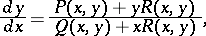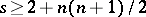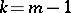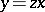# Darboux equation

(diff) ← Older revision | Latest revision (diff) | Newer revision → (diff)where,andare integral polynomials inand. This equation was first studied by G. Darboux . The Jacobi equation is a special case of the Darboux equation. Letbe a highest degree of the polynomials,,; if the Darboux equation hasknown particular algebraic solutions, then if, its general solution is found without quadratures, and if, an integrating factor can be found . Ifandare homogeneous functions of degree, andis a homogeneous function of degreethen, if, the Darboux equation is a homogeneous differential equation; if, the Darboux equation may be reduced to a Bernoulli equation by substituting.